Next: Free Electron Motion Up: Relativistic Electron Theory Previous: Dirac Equation

# Lorentz Invariance of Dirac Equation

Consider two inertial frames,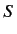and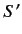. Let the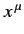and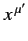be the space-time coordinates of a given event in each frame, respectively. These coordinates are related via a Lorentz transformation, which takes the general form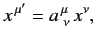(1145)

where the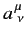are real numerical coefficients that are independent of the. We also have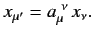(1146)

Now, since [see Equation (1102)]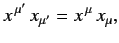(1147)

it follows that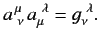(1148)

Moreover, it is easily shown that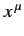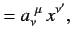(1149)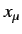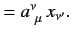(1150)

By definition, a 4-vector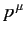has analogous transformation properties to the. Thus,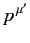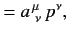(1151)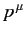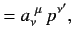(1152)

etc.

In frame, the Dirac equation is written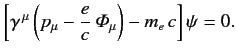(1153)

Let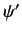be the wavefunction in frame. Suppose that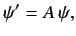(1154)

whereis a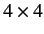transformation matrix that is independent of the. (Hence,commutes with the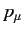and the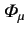.) Multiplying (1153) by, we obtain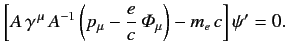(1155)

Hence, given that theandare the covariant components of 4-vectors, we obtain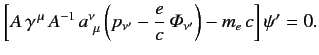(1156)

Suppose that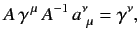(1157)

which is equivalent to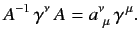(1158)

Here, we have assumed that the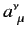commute withand the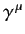(since they are just numbers). If (1157) holds then (1156) becomes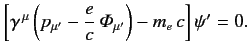(1159)

A comparison of this equation with (1153) reveals that the Dirac equation takes the same form in framesand. In other words, the Dirac equation is Lorentz invariant. Incidentally, it is clear from (1153) and (1159) that thematrices are the same in all inertial frames.

It remains to find a transformation matrixthat satisfies (1158). Consider an infinitesimal Lorentz transformation, for which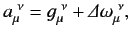(1160)

where the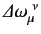are real numerical coefficients that are independent of the, and are also small compared to unity. To first order in small quantities, (1148) yields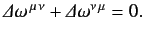(1161)

Let us write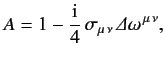(1162)

where the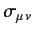are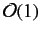matrices. To first order in small quantities,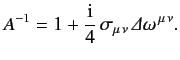(1163)

Moreover, it follows from (1161) that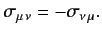(1164)

To first order in small quantities, Equations (1158), (1160), (1162), and (1163) yield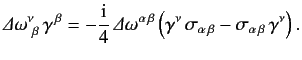(1165)

Hence, making use of the symmetry property (1161), we obtain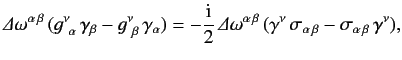(1166)

where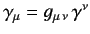. Since this equation must hold for arbitrary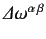, we deduce that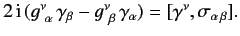(1167)

Making use of the anti-commutation relations (1122), it can be shown that a suitable solution of the above equation is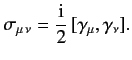(1168)

Hence,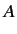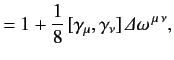(1169)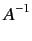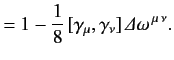(1170)

Now that we have found the correct transformation rules for an infinitesimal Lorentz transformation, we can easily find those for a finite transformation by building it up from a large number of successive infinitesimal transforms.

Making use of (1127), as well as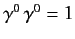, the Hermitian conjugate of (1169) can be shown to take the form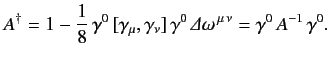(1171)

Hence, (1158) yields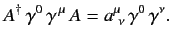(1172)

It follows that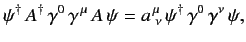(1173)

or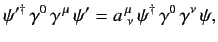(1174)

which implies that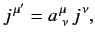(1175)

where the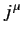are defined in Equation (1139). This proves that thetransform as the contravariant components of a 4-vector.Next: Free Electron Motion Up: Relativistic Electron Theory Previous: Dirac Equation
Richard Fitzpatrick 2013-04-08